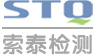中文版English设为首页加入收藏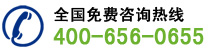新闻资讯
•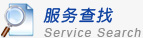•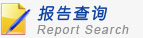•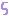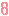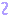•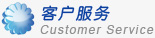•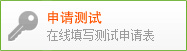•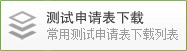•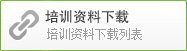•行业资讯
• 当前位置 -> 网站首页 -> 新闻资讯 -> 行业资讯

## PAHs标准更新为AfPS GS 2019:01 PAK

1) PAHs列表由原18项调整为15项，删除了苊烯/ Acenaphthylene、苊/ Acenaphthene、芴/ Fluorene三项。

2) 修改分类内容：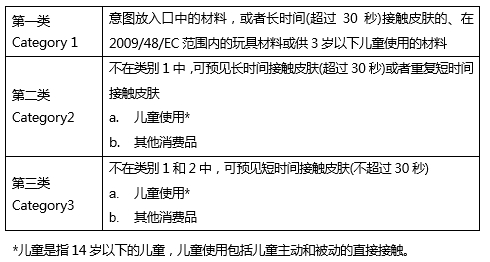项目 第一类 第二类 第三类 意图放入口中的材料或2009/48/EC中适用的玩具材料或3岁以下儿童使用且与皮肤长期接触的产品（超过30秒） 不在类别1中，可预见长时间接触皮肤(超过30秒)或者重复短时间接触皮肤 不在类别1和2中，可预见短时间接触皮肤(不超过30秒) a.儿童使用 b.其他消费品 a.儿童使用 b.其他消费品 萘 < 1 < 2 < 10 菲 总量< 1 总量< 5 总量< 10 总量< 20 总量< 50 蒽 荧蒽 芘 苯并[a]蒽 < 0.2 < 0.2 < 0.5 < 0.5 < 1 屈 < 0.2 < 0.2 < 0.5 < 0.5 < 1 苯并[b]荧蒽 < 0.2 < 0.2 < 0.5 < 0.5 < 1 苯并[k]荧蒽 < 0.2 < 0.2 < 0.5 < 0.5 < 1 苯并[a]芘 < 0.2 < 0.2 < 0.5 < 0.5 < 1 茚并[1,2,3-cd]芘 < 0.2 < 0.2 < 0.5 < 0.5 < 1 二苯并[a,h]蒽 < 0.2 < 0.2 < 0.5 < 0.5 < 1 苯并[g,h,i]芘 < 0.2 < 0.2 < 0.5 < 0.5 < 1 苯并[j]荧蒽 < 0.2 < 0.2 < 0.5 < 0.5 < 1 苯并[e]芘 < 0.2 < 0.2 < 0.5 < 0.5 < 1 15项PAHs合计 < 1 < 5 < 10 < 20 < 50

E-mail：service@stq-cert.com
•